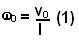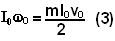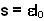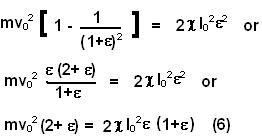## Tuesday, July 1, 2008

### Irodov Problem 1.199As soon as the mass m is given the velocity the system will start to spin about the system's CG. The CG itself will also start to move in linear motion with a velocity (m.v0+m.0)/(2m) = v0/2.

If we fix an observer at the CG however, he will see that the upper mass and lower mass are moving with a speed v0/2 in the opposite directions as shown in the figure. In other words the observer at the CG will feel as though the entire system were imparted an initial angular velocity of.

From this point onwards we shall solve the problem in the frame fixed to the CG. As the system spins around the CG, the centrifugal force will extend the spring. The moment of inertia of the system will also change as the spring extends since the masses will move further apart. Suppose that at some instant the spring is extended by a length x, then each of the masses would be at a distance x/2 from the CG. The moment of inertia of the system as a function of x will then be,Initially since x=0, the initial angular momentum of the system as seen from the CG is given by,Since there are no external torques acting on the system as seen from the CG, this angular momentum must be conserved at all times. Thus we can compute the angular velocity of the system as seen by the CG as a function of x as,As seen from the CG the masses will be subject to two kinds of motion i) rotational (the spinning) w and ii) the radial motion (masses moving towards or away from CG) vr shown in the figure. The radial motion of both masses will be equal and opposite as seen from CG since the linear momentum of the system as seen from CG must be 0, this is indicated in the figure. At maximally stretched position, however, vr will be zero.

Let the maximum stretch of the spring be x=s. The energy of the system will also be conserved at all times and so considering the initial and maximally stretched positions we have,Substitutingin (5) we have,(6) is a quadratic equation and can be solved, however, as stated in the problemis very small in comparison to 1 and so 2+= 2 and 1+=1. Thus we have,